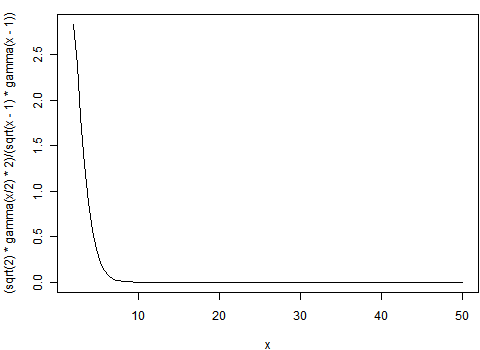# 画曲线的通用办法：描点法画图

### 谢益辉 2009-06-02

$$f(x)=(\sqrt{2}*\Gamma(x/2)*2)/(\sqrt{x-1}*\Gamma(x-1))$$

curve((sqrt(2) * gamma(x/2) * 2)/(sqrt(x - 1) * gamma(x - 1)), 2, 50)
# 当然，如果你非要按照x = seq(2, 50, ...)然后计算f(x)然后plot(x, f(x),
# type = "l")那也可以，只不过curve()就是干这事的，不必麻烦分两步走了“武功再高，也怕菜刀”绝对是真理，哈哈。